# R S Aggarwal Solutions for Class 10 Maths Chapter 7 Triangles Exercise 7B

R S Aggarwal Solutions for class 10 Maths chapter 7 exercise 7B is available here. These solutions are prepared by subject experts at BYJU’S to help students clear their doubts. Students can freely download R S Aggarwal Class 10 chapter 7 from our website.

## Download PDF of R S Aggarwal Solutions for Class 10 Maths Chapter 7 Triangles Exercise 7B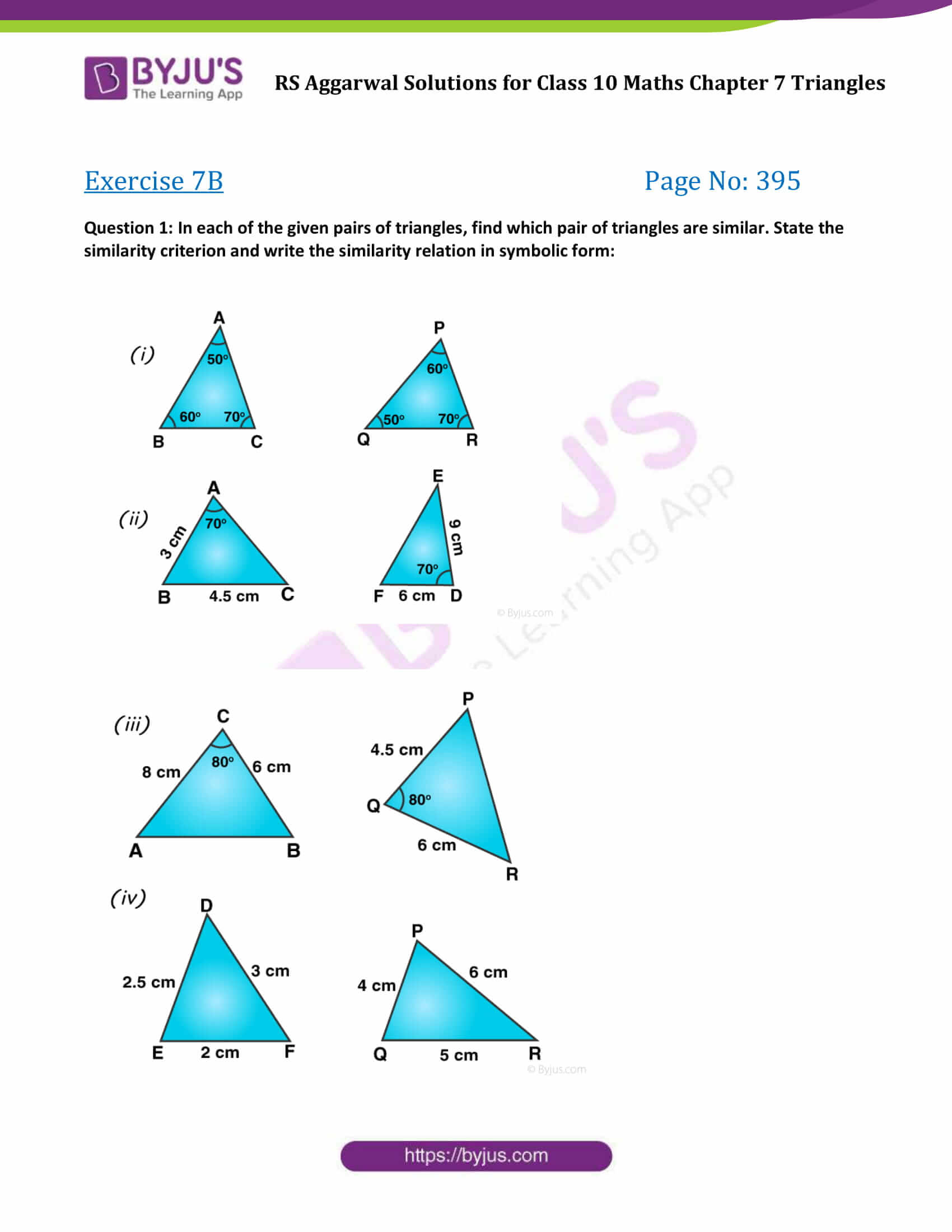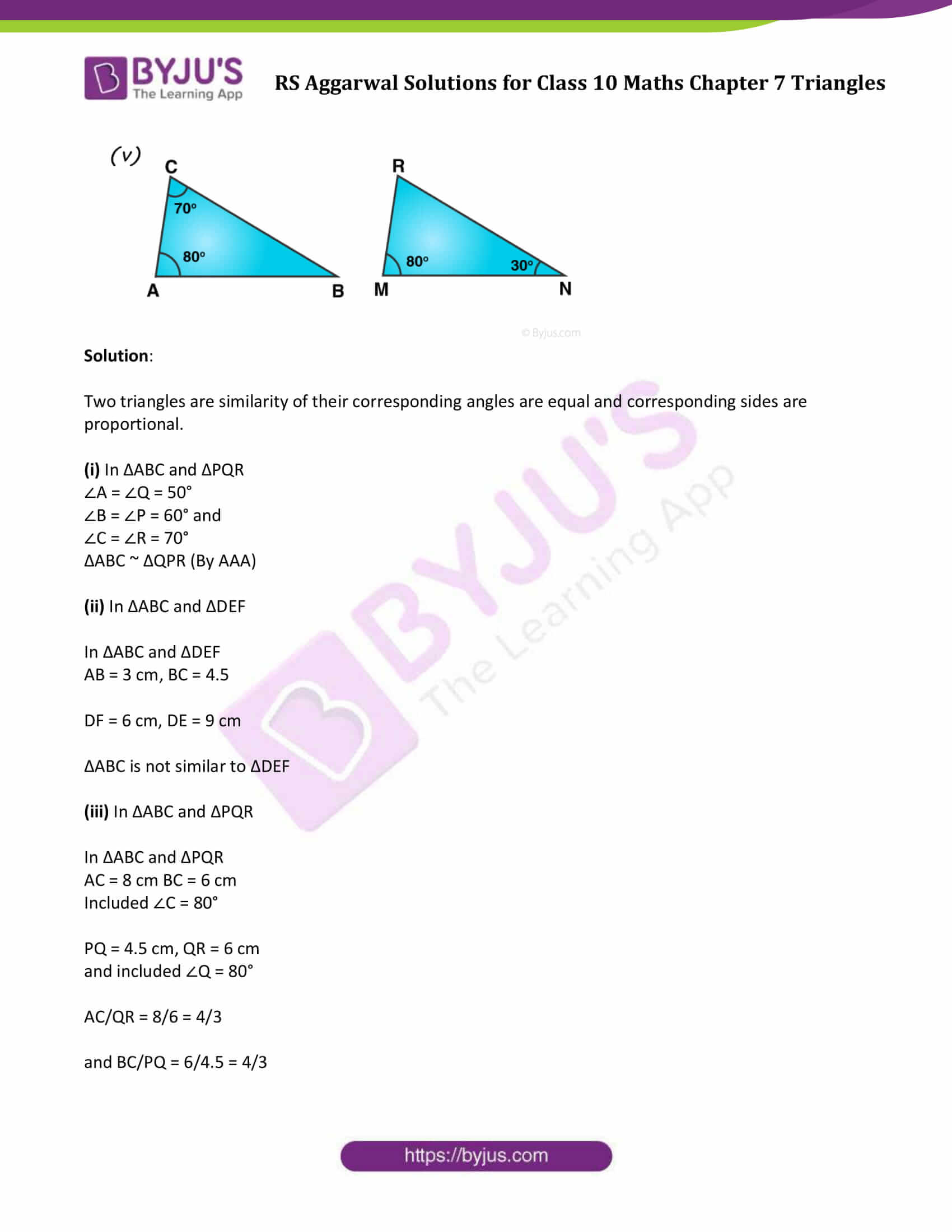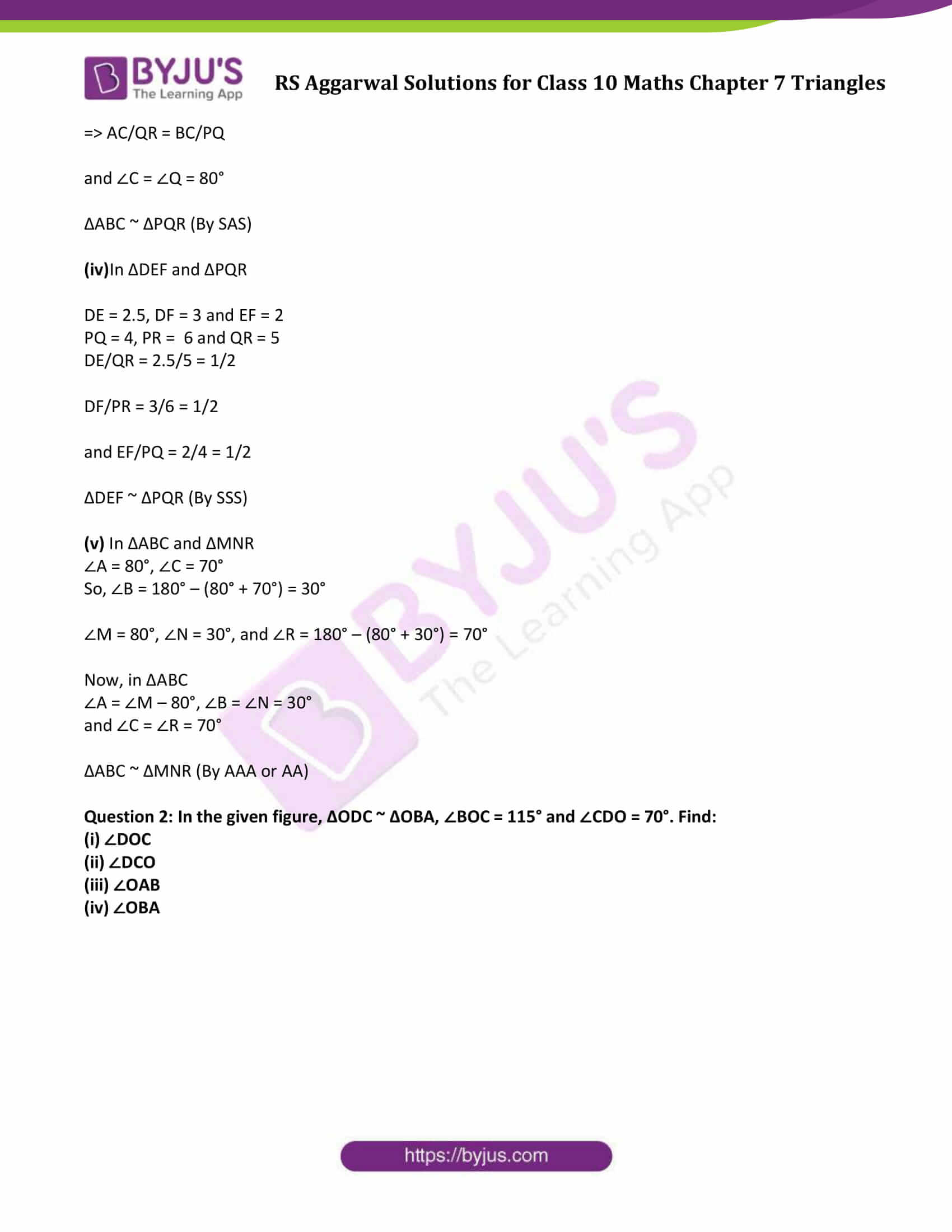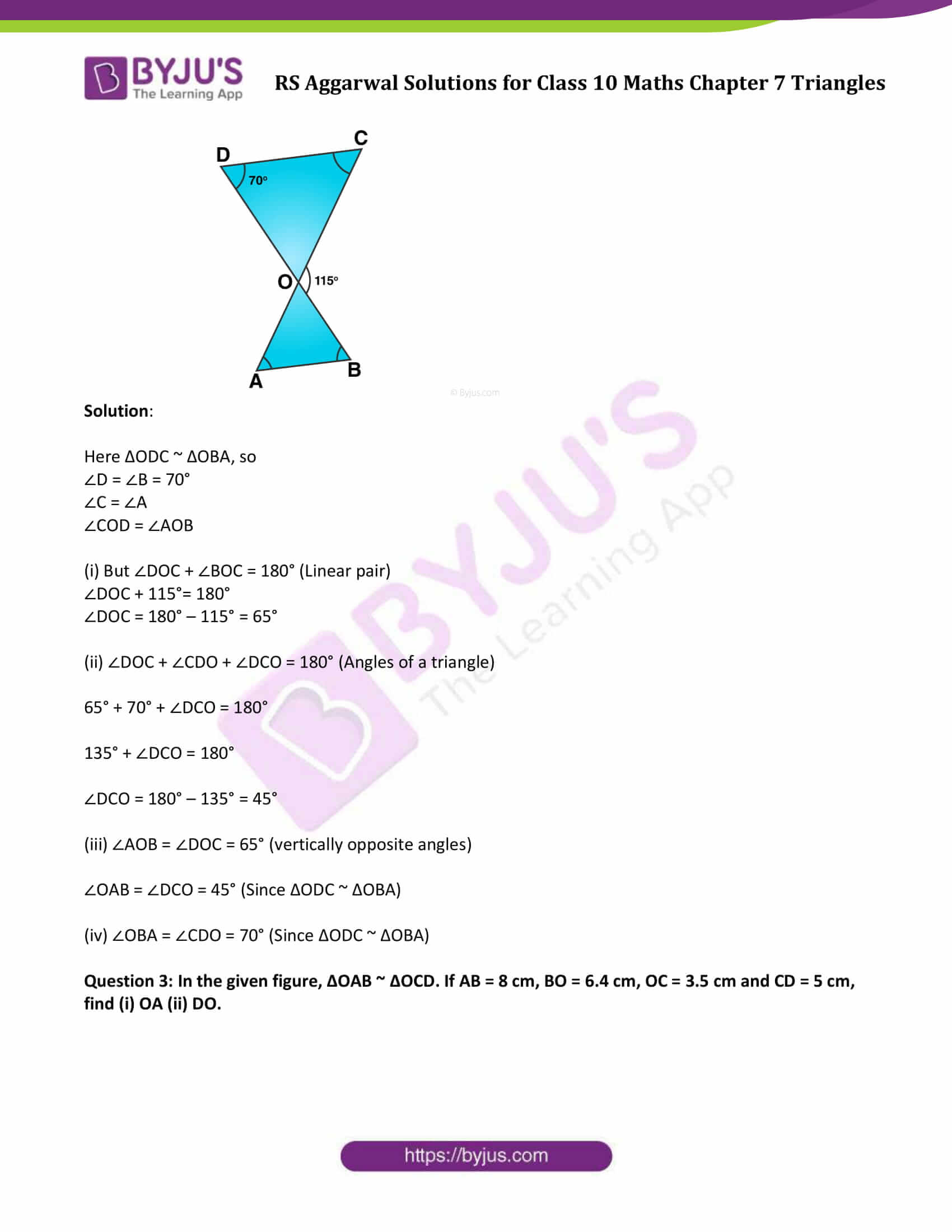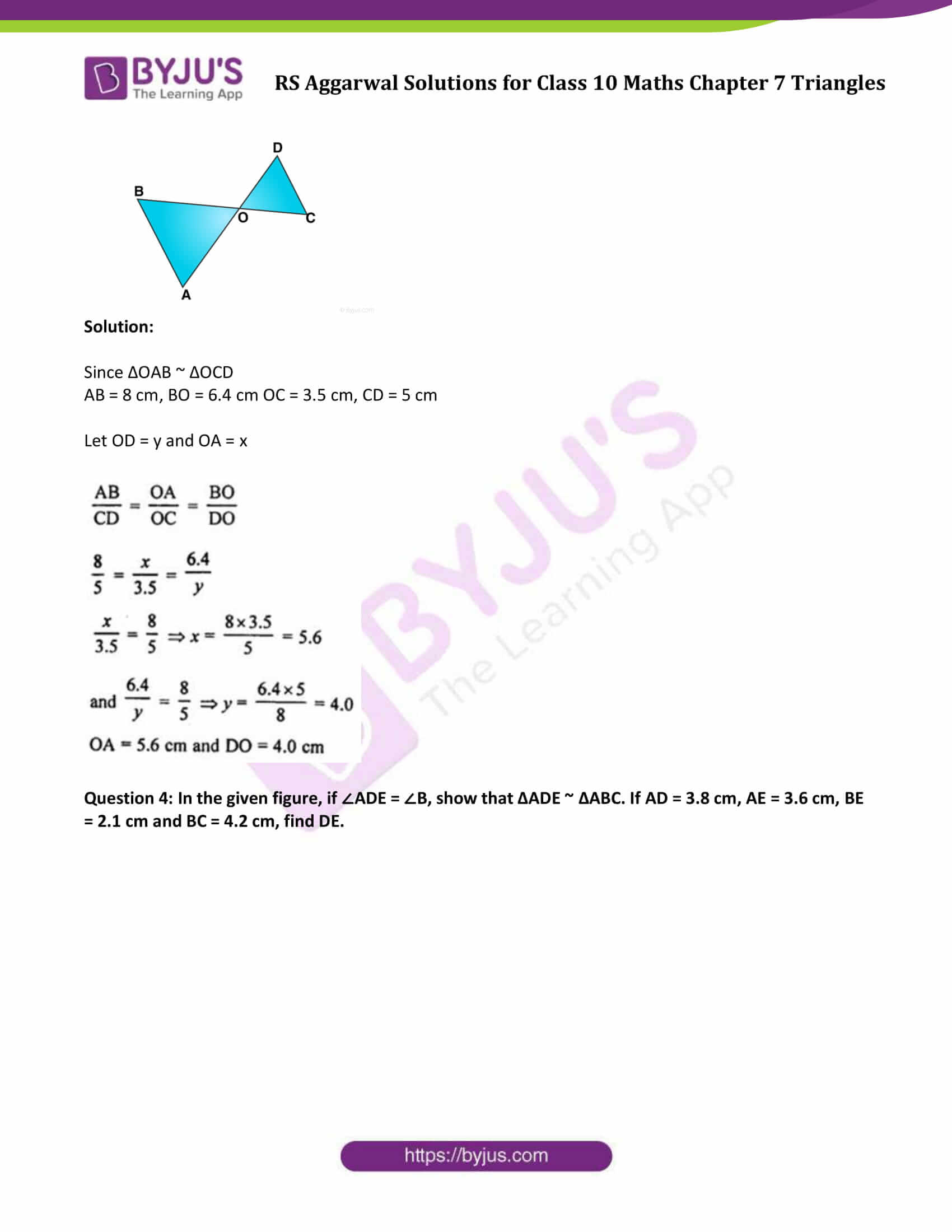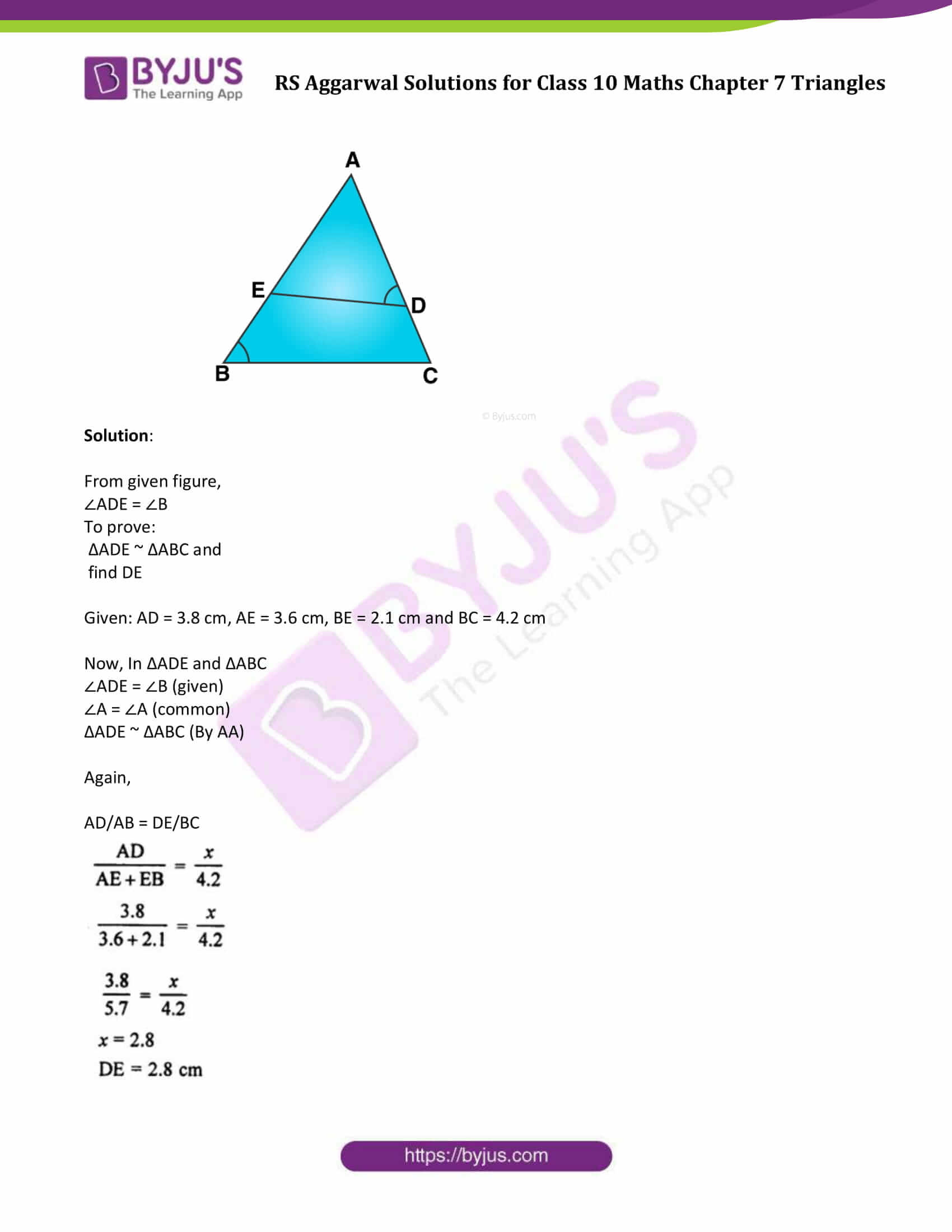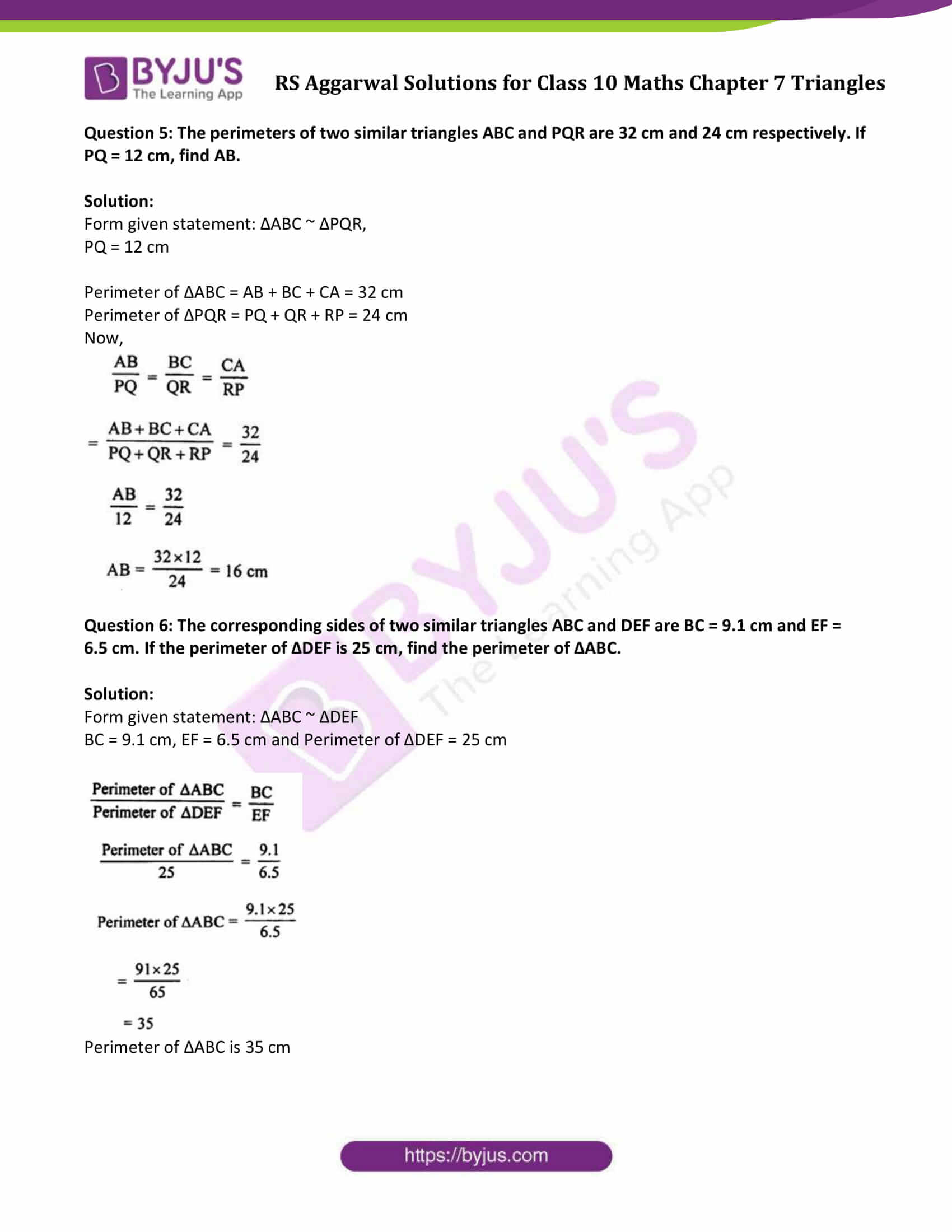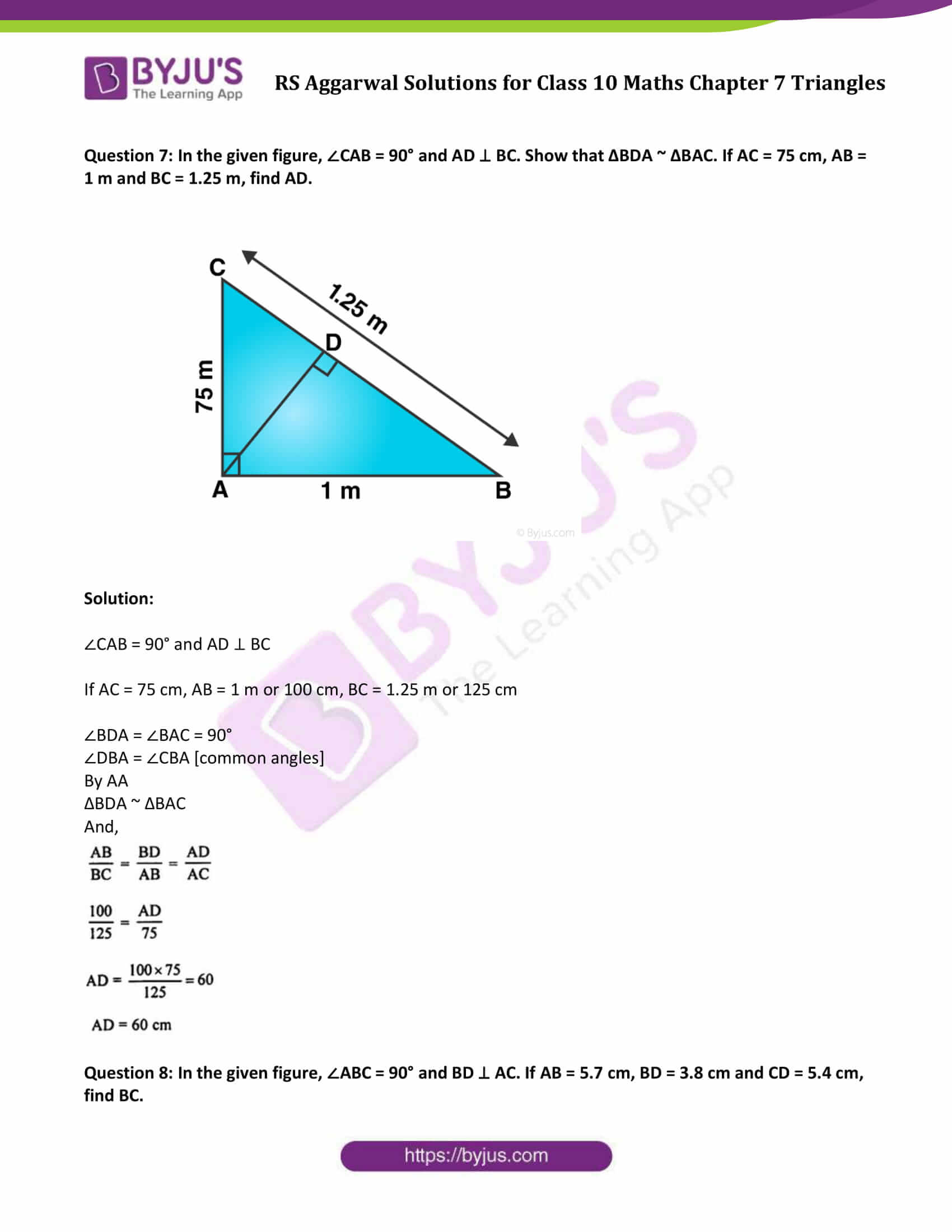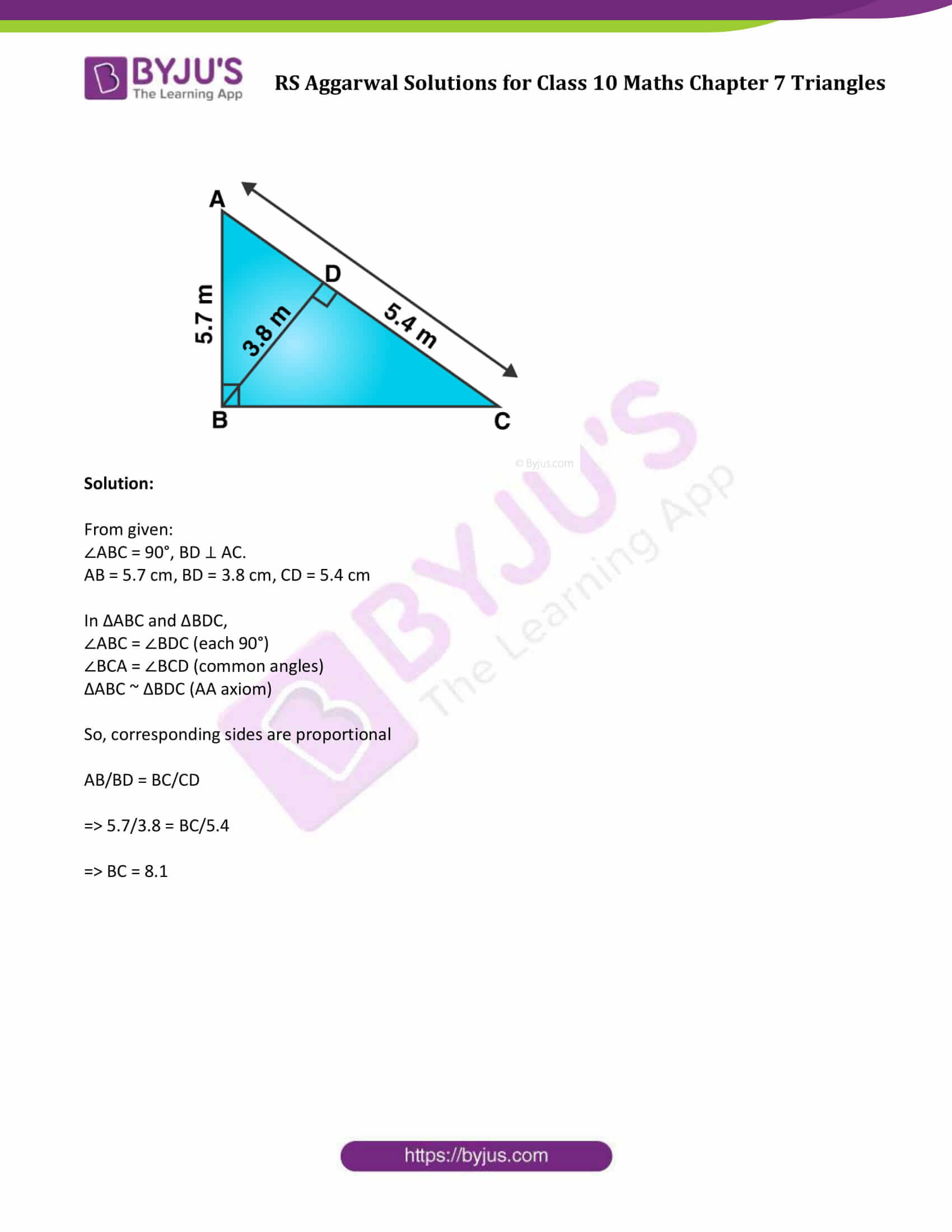### Access other exercise solutions of Class 10 Maths Chapter 7 Triangles

Exercise 7 A Solutions

Exercise 7 C Solutions

Exercise 7 D Solutions

Exercise 7 E Solutions

Question 1: In each of the given pairs of triangles, find which pair of triangles are similar. State the similarity criterion and write the similarity relation in symbolic form: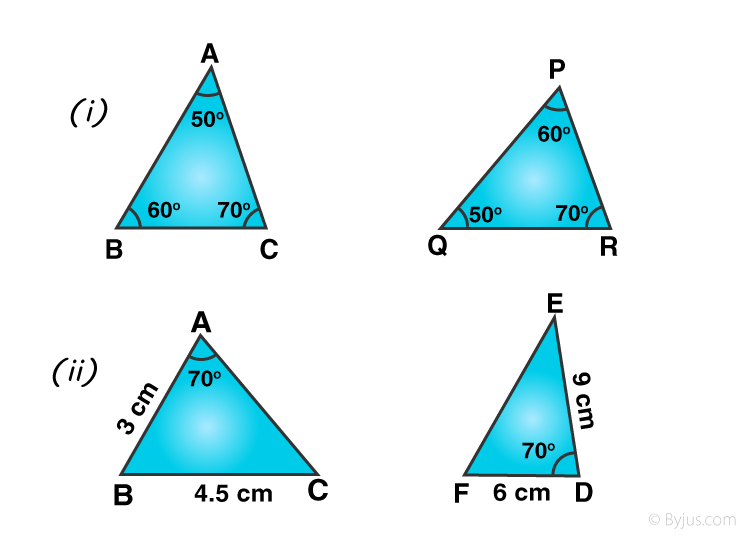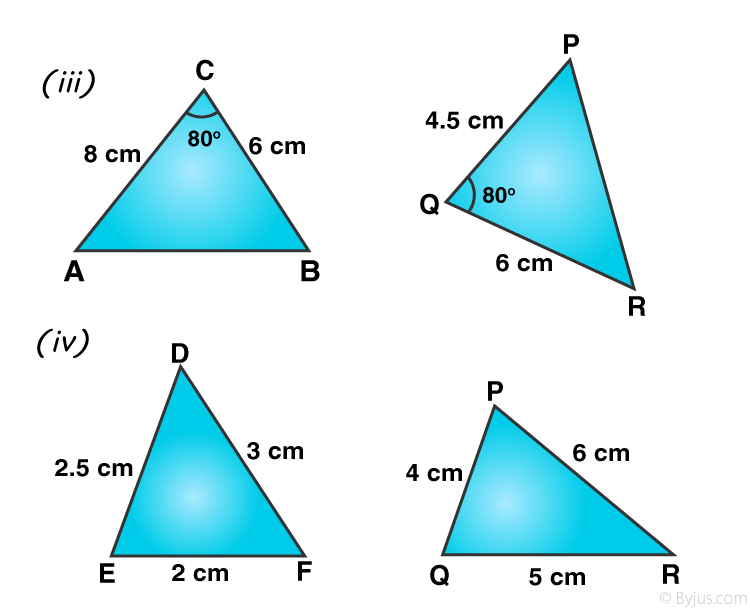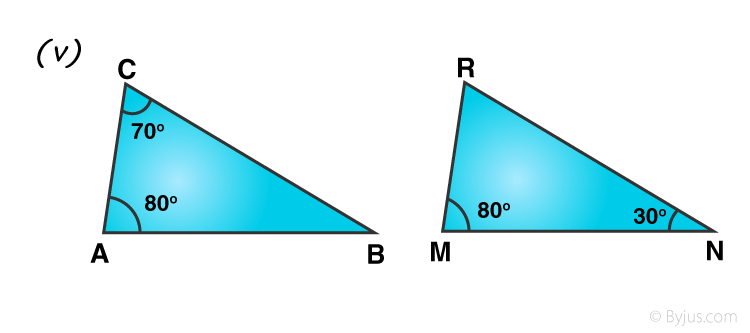Solution:

Two triangles are similarity of their corresponding angles are equal and corresponding sides are proportional.

(i) In ∆ABC and ∆PQR

∠A = ∠Q = 50°

∠B = ∠P = 60° and

∠C = ∠R = 70°

∆ABC ~ ∆QPR (By AAA)

(ii) In ∆ABC and ∆DEF

In ∆ABC and ∆DEF

AB = 3 cm, BC = 4.5

DF = 6 cm, DE = 9 cm

∆ABC is not similar to ∆DEF

(iii) In ∆ABC and ∆PQR

In ∆ABC and ∆PQR

AC = 8 cm BC = 6 cm

Included ∠C = 80°

PQ = 4.5 cm, QR = 6 cm

and included ∠Q = 80°

AC/QR = 8/6 = 4/3

and BC/PQ = 6/4.5 = 4/3

=> AC/QR = BC/PQ

and ∠C = ∠Q = 80°

∆ABC ~ ∆PQR (By SAS)

(iv)In ∆DEF and ∆PQR

DE = 2.5, DF = 3 and EF = 2

PQ = 4, PR = 6 and QR = 5

DE/QR = 2.5/5 = 1/2

DF/PR = 3/6 = 1/2

and EF/PQ = 2/4 = 1/2

∆DEF ~ ∆PQR (By SSS)

(v) In ∆ABC and ∆MNR

∠A = 80°, ∠C = 70°

So, ∠B = 180° – (80° + 70°) = 30°

∠M = 80°, ∠N = 30°, and ∠R = 180° – (80° + 30°) = 70°

Now, in ∆ABC

∠A = ∠M – 80°, ∠B = ∠N = 30°

and ∠C = ∠R = 70°

∆ABC ~ ∆MNR (By AAA or AA)

Question 2: In the given figure, ∆ODC ~ ∆OBA, ∠BOC = 115° and ∠CDO = 70°. Find:

(i) ∠DOC

(ii) ∠DCO

(iii) ∠OAB

(iv) ∠OBA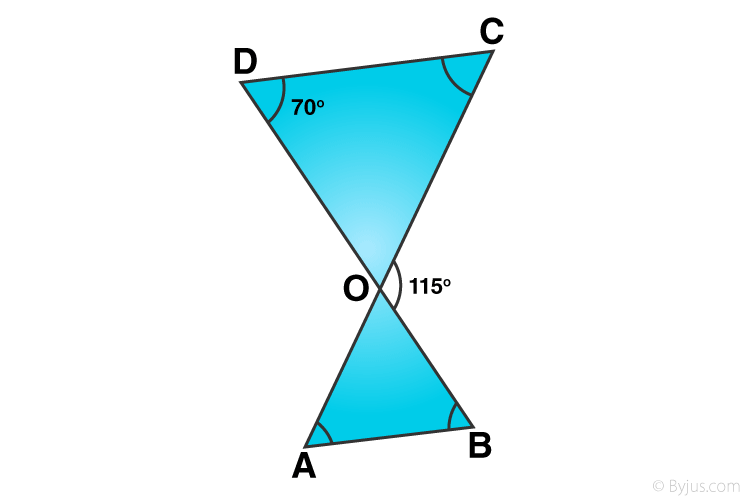Solution:

Here ∆ODC ~ ∆OBA, so

∠D = ∠B = 70°

∠C = ∠A

∠COD = ∠AOB

(i) But ∠DOC + ∠BOC = 180° (Linear pair)

∠DOC + 115°= 180°

∠DOC = 180° – 115° = 65°

(ii) ∠DOC + ∠CDO + ∠DCO = 180° (Angles of a triangle)

65° + 70° + ∠DCO = 180°

135° + ∠DCO = 180°

∠DCO = 180° – 135° = 45°

(iii) ∠AOB = ∠DOC = 65° (vertically opposite angles)

∠OAB = ∠DCO = 45° (Since ∆ODC ~ ∆OBA)

(iv) ∠OBA = ∠CDO = 70° (Since ∆ODC ~ ∆OBA)

Question 3: In the given figure, ∆OAB ~ ∆OCD. If AB = 8 cm, BO = 6.4 cm, OC = 3.5 cm and CD = 5 cm, find (i) OA (ii) DO.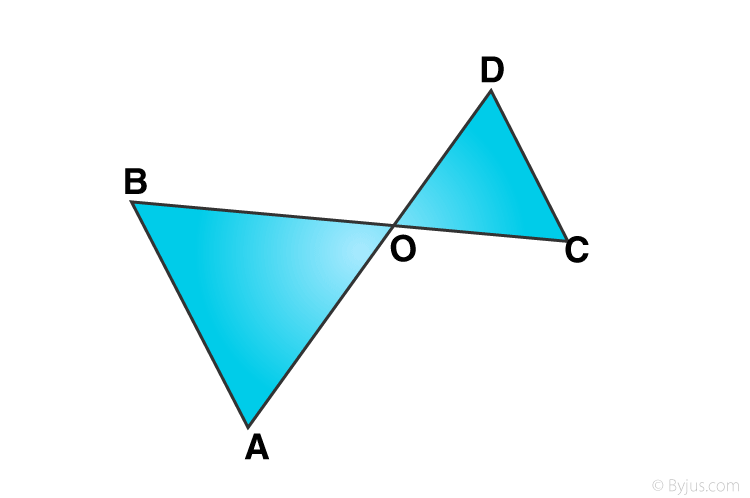Solution:

Since ∆OAB ~ ∆OCD

AB = 8 cm, BO = 6.4 cm OC = 3.5 cm, CD = 5 cm

Let OD = y and OA = x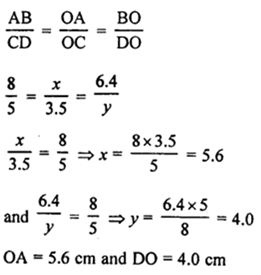Question 4: In the given figure, if ∠ADE = ∠B, show that ∆ADE ~ ∆ABC. If AD = 3.8 cm, AE = 3.6 cm, BE = 2.1 cm and BC = 4.2 cm, find DE.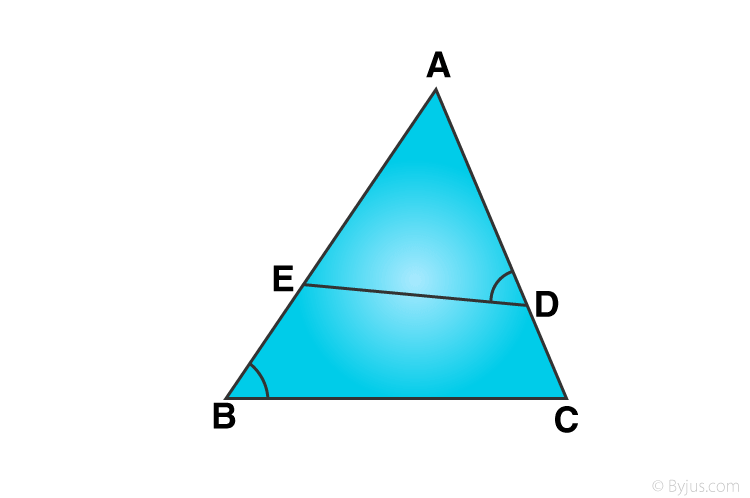Solution:

From given figure,

To prove:

find DE

Given: AD = 3.8 cm, AE = 3.6 cm, BE = 2.1 cm and BC = 4.2 cm

∠A = ∠A (common)

Again,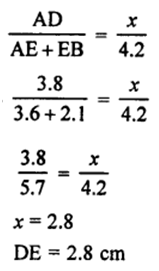Question 5: The perimeters of two similar triangles ABC and PQR are 32 cm and 24 cm respectively. If PQ = 12 cm, find AB.

Solution:

Form given statement: ∆ABC ~ ∆PQR,

PQ = 12 cm

Perimeter of ∆ABC = AB + BC + CA = 32 cm

Perimeter of ∆PQR = PQ + QR + RP = 24 cm

Now,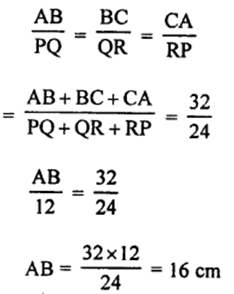Question 6: The corresponding sides of two similar triangles ABC and DEF are BC = 9.1 cm and EF = 6.5 cm. If the perimeter of ∆DEF is 25 cm, find the perimeter of ∆ABC.

Solution:

Form given statement: ∆ABC ~ ∆DEF

BC = 9.1 cm, EF = 6.5 cm and Perimeter of ∆DEF = 25 cm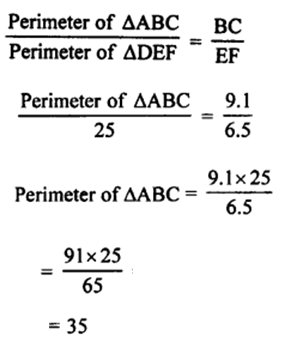Perimeter of ∆ABC is 35 cm

Question 7: In the given figure, ∠CAB = 90° and AD ⊥ BC. Show that ∆BDA ~ ∆BAC. If AC = 75 cm, AB = 1 m and BC = 1.25 m, find AD.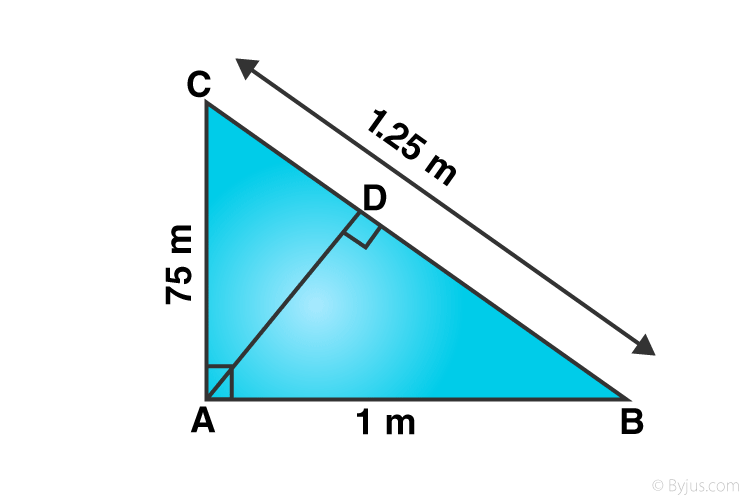Solution:

∠CAB = 90° and AD ⊥ BC

If AC = 75 cm, AB = 1 m or 100 cm, BC = 1.25 m or 125 cm

∠BDA = ∠BAC = 90°

∠DBA = ∠CBA [common angles]

By AA

∆BDA ~ ∆BAC

And,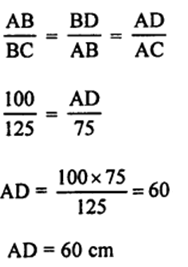Question 8: In the given figure, ∠ABC = 90° and BD ⊥ AC. If AB = 5.7 cm, BD = 3.8 cm and CD = 5.4 cm, find BC.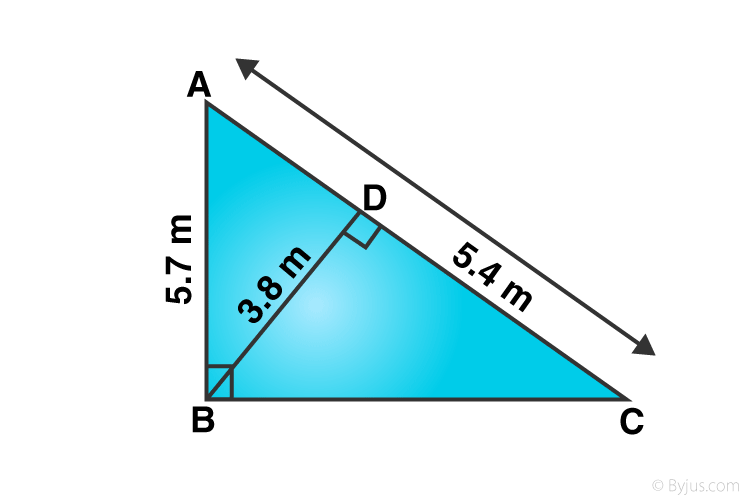Solution:

From given:

∠ABC = 90°, BD ⊥ AC.

AB = 5.7 cm, BD = 3.8 cm, CD = 5.4 cm

In ∆ABC and ∆BDC,

∠ABC = ∠BDC (each 90°)

∠BCA = ∠BCD (common angles)

∆ABC ~ ∆BDC (AA axiom)

So, corresponding sides are proportional

AB/BD = BC/CD

=> 5.7/3.8 = BC/5.4

=> BC = 8.1

## R S Aggarwal Solutions for Class 10 Maths Chapter 7 Triangles Exercise 7B

Class 10 Maths Chapter 7 Triangles Exercise 7B is based on criteria for the similarity of two triangles

• AAA similarity
• AA similarity
• SSS similarity
• SAS similarity